### CAT 2022 Question Paper (Slot 3) Question 43

Instructions

Pulak, Qasim, Ritesh, and Suresh participated in a tournament comprising of eight rounds. In each round, they formed two pairs, with each of them being in exactly one pair. The only restriction in the pairing was that the pairs would change in successive rounds. For example, if Pulak formed a pair with Qasim in the first round, then he would have to form a pair with Ritesh or Suresh in the second round. He would be free to pair with Qasim again in the third round. In each round, each pair decided whether to play the game in that round or not. If they decided not to play, then no money was exchanged between them. If they decided to play, they had to bet either ₹1 or ₹2 in that round. For example, if they chose to bet ₹2, then the player winning the game got ₹2 from the one losing the game.

At the beginning of the tournament, the players had ₹10 each. The following table shows partial information about the amounts that the players had at the end of each of the eightrounds. It shows every time a player had ₹10 at the end of a round, as well as every time, at the end of a round, a player had either the minimum or the maximum amount that he would have had across the eight rounds. For example, Suresh had ₹10 at the end of Rounds 1, 3 and 8 and not after any of the other rounds. The maximum amount that he had at the end of any round was ₹13 (at the end of Round 5), and the minimum amount he had at the end of any round was ₹8 (at the end of Round 2). At the end of all other rounds, he must have had either ₹9, ₹11, or ₹12.

It was also known that Pulak and Qasim had the same amount of money with them at the end of Round 4.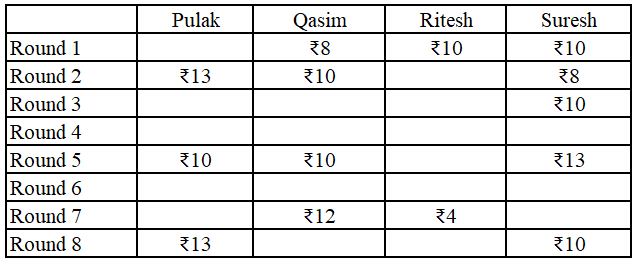Question 43

# How many games were played with a bet of ₹2?

Solution

Its given that the table shows every time a player had ₹10 at the end of a round, as well as every time, at the end of a round, a player had either the minimum or the maximum amount that he would have had across the eight rounds, which means that in Pulak's column the numbers possible are 11 or 12 only, similarly in Qasim's column numbers possible are only 9 or 11 only, similarly in Ritesh's column numbers possible are 5,6,7,8 or 9 only and in Suresh's column numbers possible are 9,11 or 12 only.

Everyone started with 10 rupees and the total amount,i.e., 40 in each round remains the same. So we get the following table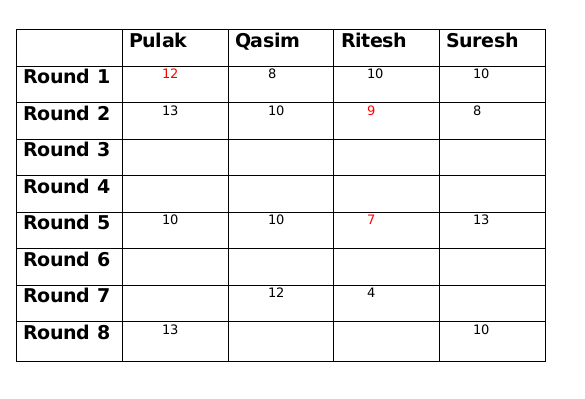We are given that at the end of 4th round both Pulak and Qasim had the same amount with them and that amount possible is only 11.

As per the information given in the set the only possible amount with Qasim at the end of round 6 is 11.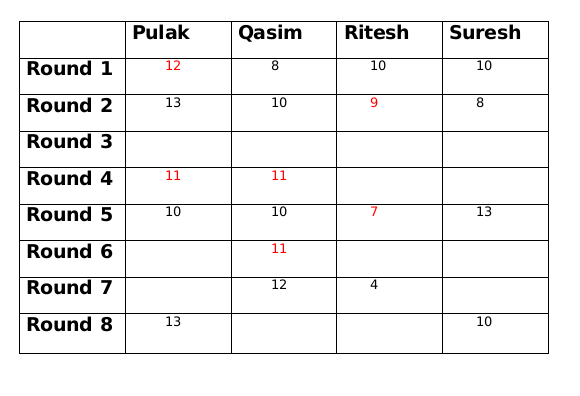Amounts with Pulak and Qasim at the end of round 5 is decreased by 1 from the amounts which they had at the end of round 4, since the total amount will be same at the end of every round, so amounts with Ritesh and Suresh at the end of round 5 is increased by 1 from the amounts which they had at the end of round 4.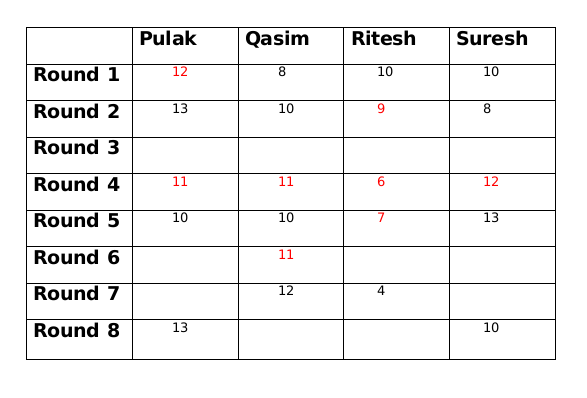Amount with Suresh at the end of round 2 is 8 and at the end of round 4 is 12, therefore, amount with Suresh at the end of round 3 is 10.

Amount with Qasim at the end of round 3 is either 9 or 11. If the amount is 9 then either amount with Ritesh has to be 10 or amount with Pulak has to to be 14, both of which is not possible. Therefore, amount with Qasim at the end of round 3 is 11.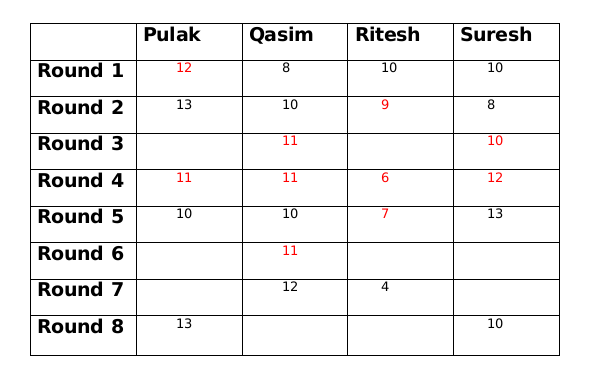Now Qasim has the same amount at the end of round 3 and round 4 so there must be another person whose amount is also same at the end of round 3 and round 4. This person can only be Pulak. So at the end of round 3 Pulak has 11 rupees and Ritesh has 8 rupees.

The only possible amount with Qasim at the end of round 8 can be 11 and therefore the amount at the end of round 8 with Ritesh will be 6.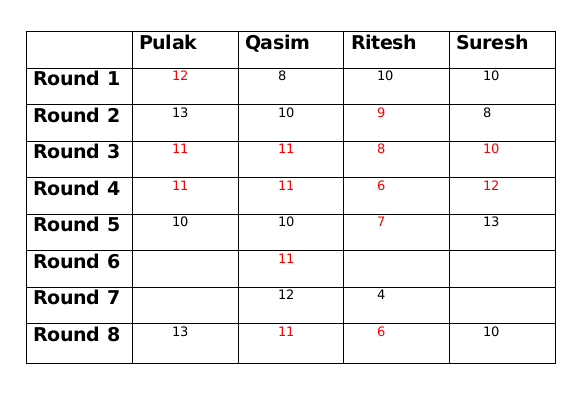The amount with Ritesh at the end of round 8 is 2 more than the amount with him at the end of round 7, so the amount with either Pulak or Suresh at the end of round 7 has to be 2 more than the amount at the end of round 8. This is only possible for Suresh who must have 12 rupees at the end of round 7 as Pulak cannot have rupees 15 at the end of round 7.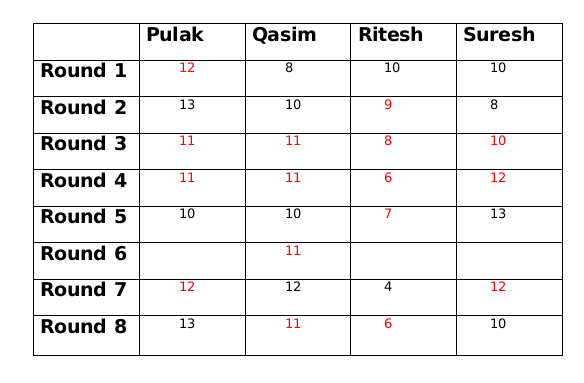The amount with Qasim at the end of round 7 is 1 more than the amount with him at the end of round 6. So there must be another person whose amount at the end of round 7 is 1 less than the amount him at the end of round 6. This only possible person can be Ritesh. So amount with Ritesh at the end of round 6 will be 5.

If the amount with Suresh at the end of round 6 is 11 then the amount with Pulak at the end of round 6 will be 13 which is not possible. Therefore the amount with Suresh at the end of round 6 is 12 and the amount with Pulak at the end of round 6 is 12.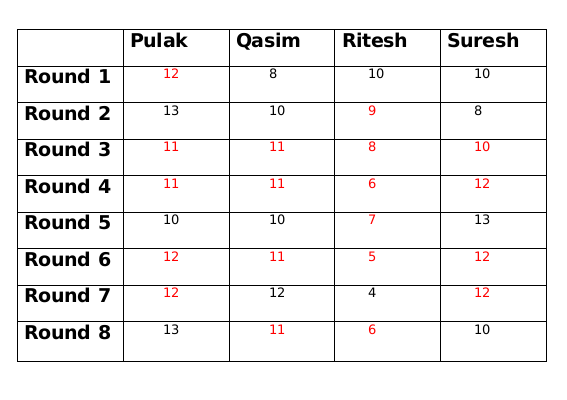The Games which had a bet of Rs.2 are as following
Round 1 - Palak vs Qasim
Round 2 - Qasim vs Suresh
Round 3 - Palak vs Suresh
Round 4 - Ritesh vs Suresh
Round 5 - None
Round 6 - Palak vs Ritesh
Round 7 - None
Round 8 - Ritesh vs Suresh
Hence total number of games that were played with a bet of 2 is 6